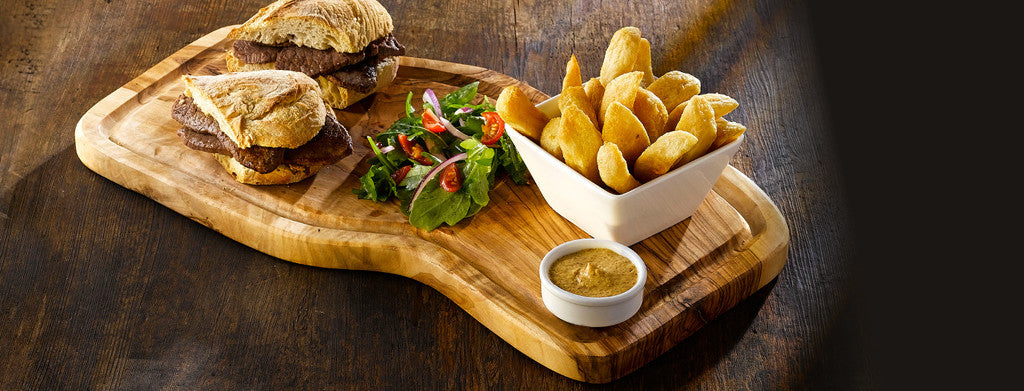Shopping Cart

### Refine

View all

#### Price# Tabletop Presentation£16.00 each (ex VAT)£13.00 each (ex VAT)£8.73 each (ex VAT)£11.56 each (ex VAT)£13.98 each (ex VAT)£2.57 each (ex VAT)£9.81 each (ex VAT)£7.30 each (ex VAT)£8.30 each (ex VAT)£7.00 each (ex VAT)£7.70 each (ex VAT)£9.40 each (ex VAT)£3.06 each (ex VAT)£1.40 each (ex VAT)£12.96 each (ex VAT)£13.56 each (ex VAT)£25.30 each (ex VAT)£36.76 each (ex VAT)£17.12 each (ex VAT)£21.60 each (ex VAT)£29.78 each (ex VAT)£19.46 each (ex VAT)£17.94 each (ex VAT)£22.20 each (ex VAT)£20.98 each (ex VAT)£6.78 each (ex VAT)£7.30 each (ex VAT)£7.86 each (ex VAT)£9.94 each (ex VAT)£12.82 each (ex VAT)£15.62 each (ex VAT)£10.14 each (ex VAT)£10.14 each (ex VAT)£1.94 each (ex VAT)£2.24 each (ex VAT)£8.10 each (ex VAT)£8.10 each (ex VAT)£6.06 each (ex VAT)£6.38 each (ex VAT)£6.38 each (ex VAT)£6.38 each (ex VAT)£4.88 each (ex VAT)£5.56 each (ex VAT)£6.40 each (ex VAT)£3.82 each (ex VAT)£4.02 each (ex VAT)£5.80 each (ex VAT)£6.62 each (ex VAT)